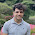## Problem:

In the 5 by 5 matrix below, the minimal path sum from the top left to the bottom right, by only moving to the right and down, is indicated in bold red and is equal to 2427.

131 673 234 103 18
201 96 342 965 150
630 803 746 422 111
537 699 497 121 956
805 732 524 37 331

Find the minimal path sum, in matrix.txt (right click and 'Save Link/Target As...'), a 31K text file containing a 80 by 80 matrix, from the top left to the bottom right by only moving right and down.

73682

## Code:The solution may include methods that will be found here: Library.java .

public interface EulerSolution{public String run();}
/*  * Solution to Project Euler problem 31 * By Nayuki Minase *  * http://nayuki.eigenstate.org/page/project-euler-solutions * https://github.com/nayuki/Project-Euler-solutions */public final class p031 implements EulerSolution {		public static void main(String[] args) {		System.out.println(new p031().run());	}			private static final int TOTAL = 200;	private static int[] COINS = {1, 2, 5, 10, 20, 50, 100, 200};		public String run() {		// Knapsack problem. ways[i][j] is the number of ways to use		// any of the first i coin values to form an unordered sum of j.		int[][] ways = new int[COINS.length + 1][TOTAL + 1];		ways = 1;		for (int i = 0; i < COINS.length; i++) {			for (int j = 0; j <= TOTAL; j++)				ways[i + 1][j] = ways[i][j] + (j >= COINS[i] ? ways[i + 1][j - COINS[i]] : 0);  // Dynamic programming		}		return Integer.toString(ways[COINS.length][TOTAL]);	}	}

#### 2 comments :

1.Most of the programs have been copied from github repo of a person; and yet no credit or reference has been given to that person.

Show some respect buddy.

1.Ignorant dummie. Look at the comment section at the beginning of the code, you will see it being attributed to Nayuki Minase.

## Follow Me

If you like our content, feel free to follow me to stay updated.

## Subscribe

Enter your email address:

We hate spam as much as you do.

## Upload Material

Got an exam, project, tutorial video, exercise, solutions, unsolved problem, question, solution manual? We are open to any coding material. Why not upload?
Copyright © 2012 - 2014 Java Problems  --  About  --  Attribution  --  Privacy Policy  --  Terms of Use  --  Contact